Courses

# GOC & Aromaticity - 2

## 20 Questions MCQ Test GATE Chemistry Mock Test Series | GOC & Aromaticity - 2

Description
This mock test of GOC & Aromaticity - 2 for Chemistry helps you for every Chemistry entrance exam. This contains 20 Multiple Choice Questions for Chemistry GOC & Aromaticity - 2 (mcq) to study with solutions a complete question bank. The solved questions answers in this GOC & Aromaticity - 2 quiz give you a good mix of easy questions and tough questions. Chemistry students definitely take this GOC & Aromaticity - 2 exercise for a better result in the exam. You can find other GOC & Aromaticity - 2 extra questions, long questions & short questions for Chemistry on EduRev as well by searching above.
QUESTION: 1

### The Correct order of pKa  value of the follwing compound will be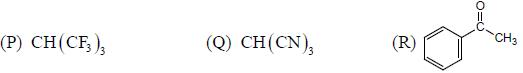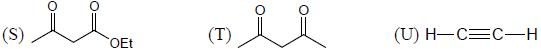Solution:

As we know that acidic b ehaviour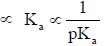Then the least acidic com pound will have highest pKa.  Here, conjugate base of Q will be most stable and thus, will be most acidic and thus Q will be most stable and thus, will be most acidic and thus Q have lowest pKa . On the other hand T will be more acidic as compared to S. because S involves cross conjugation. U will be least acidic. Thus, order of pKa value will be
Q < T < S < P < R < U

QUESTION: 2

### The correct order of acidic nature of following compound is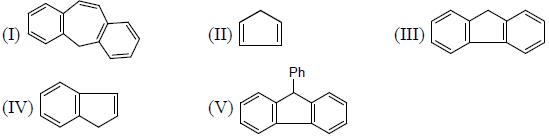Solution:

Conjugate base of I, II. Ill, IV and V are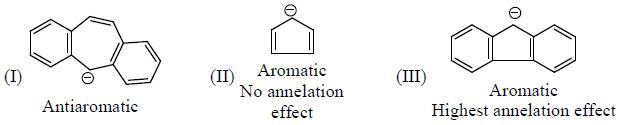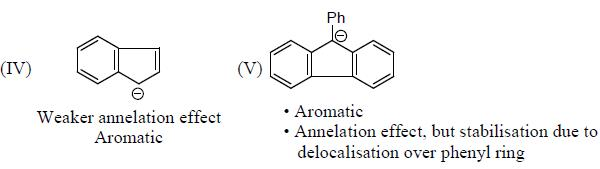Here. II will be the most stable because of extensive delocalization and equal contributing resonance structure, followed by V in which negative charge is delocalized over additional phenyl ring.
Thus, acidity order will be
II > V > IV > III > I

QUESTION: 3

### The acidic behaviour of the following substituted benzoic acid will be in the order of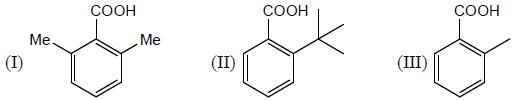Solution:

Electron donating group decreases acidic behaviour.
• For hyper conjugation and resonance to happen, the overlapping atom must be planer
• The moment, there is deviation from planarity, because of any reason (here because of steric repulsion) the said effect will minimize or will be diminished.
Becuase of steric effect least donation will be in I, thus will be most acidic Hence, acidic order will be I > II > III.

QUESTION: 4

The correct order for the rates of electrophilic aromatic substitution of the following compound is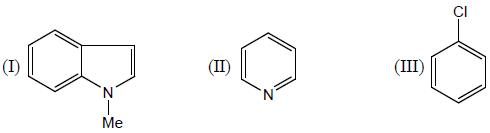Solution:

Rate of electrophilic aromatic substitution reaction depends upon availability of electron cloud
• Effect of an atom as an electron withdrawing group will be more, when it will be present in the ring, as compared to when it will present outside the ring. Thus, the rate of EAS will be I > III > II. Hence, option (d) is correct.

QUESTION: 5

Among the bromide l-lll given below, the order of their reactivity in the SN1 reaction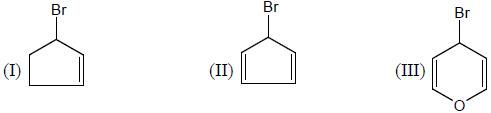Solution:

Rate of SN1 reaction ∝ stability of carbocation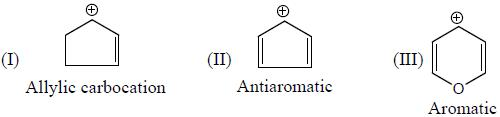Thus, stability order will be III > I > II
Hence, rate of SN1 will also be in same order III > I > II

QUESTION: 6

Choose the incorrect statement about tropolone

Solution: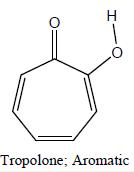it exist predominately in the enol form, in which O-H bond is considerably polar, hence have high dipole moment.
Since, it predominately exist in enol form. It gives reaction corresponding to phenol and not corresponding to ketone. That is why it given FeClj test as well as Reimer Tiemann reaction, but does not gives DNP test.

QUESTION: 7

Among the following molecule. The most acidic molecule is: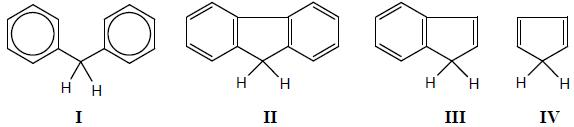Solution: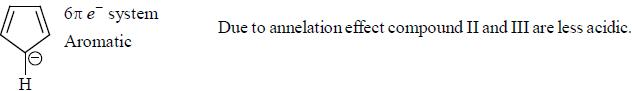QUESTION: 8

The decreasing order ofnucleophilicity for the following anions is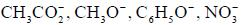Solution: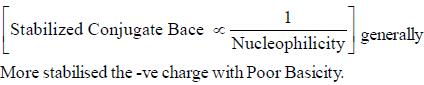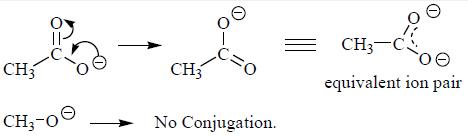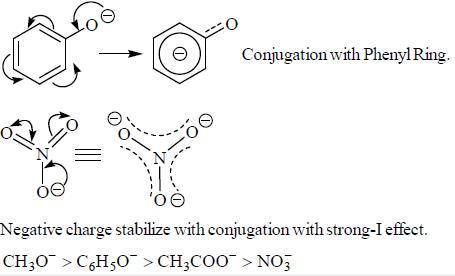*Answer can only contain numeric values
QUESTION: 9

How many compound(s) will not rotate the plane polarized light.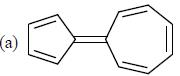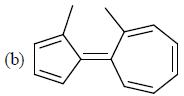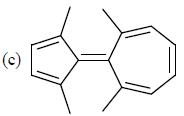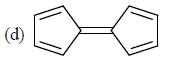Solution: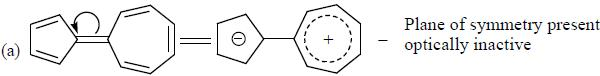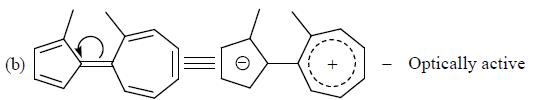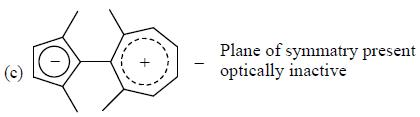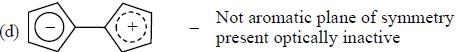*Answer can only contain numeric values
QUESTION: 10

Number of non-aromatic compound is / are ___________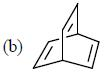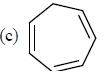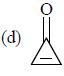Solution: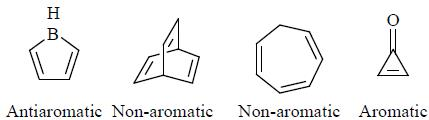QUESTION: 11

The increasing order of acidity of the following molecules.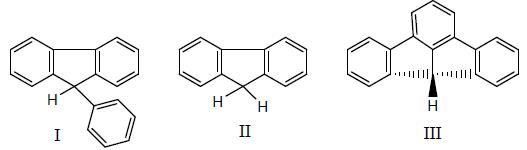Solution: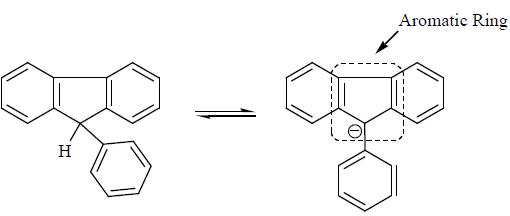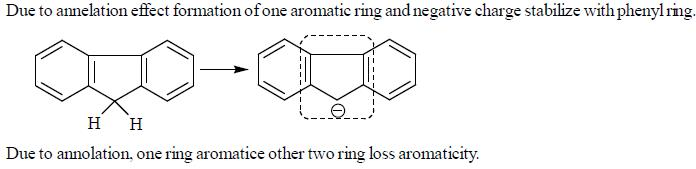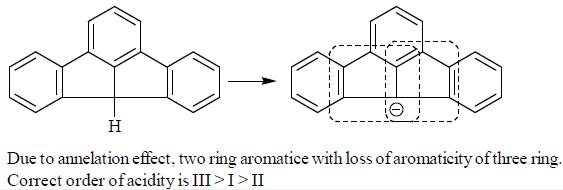QUESTION: 12

The collect order of increasing reactivity towards nucleophilic addition for the following carbonyl derivative.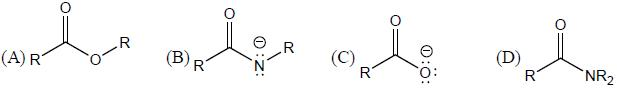Solution:

Reactivity towards nucleophile means electrophilicity. Electrophilic character at Carbonyl carbon depends upon the neighbouring group. Electron releasing group decrease electrophilicity and electron withdrawing group increase electrophilicity. Negative charge containing atom are better donar as compared to lone pair containing atom. Less electronegative atom (N) is better donar as compared to more electronegative atom (O). So electrophilic order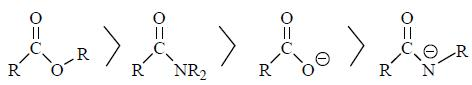QUESTION: 13

Which one of the following has highest pKb ?

Solution: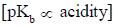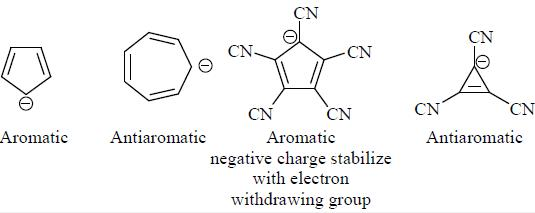QUESTION: 14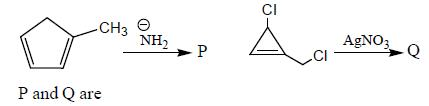Solution: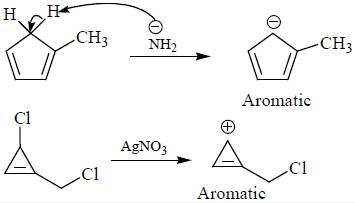QUESTION: 15

Dipole moment of which ketone is maximum ?

Solution: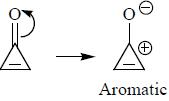QUESTION: 16

Incorrect order of acidity

Solution:

More stabilized conjugate base with more acidity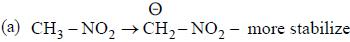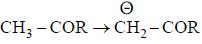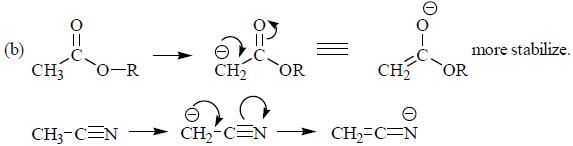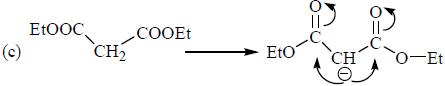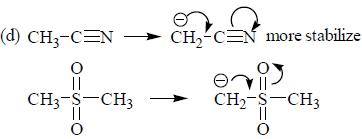QUESTION: 17

Pickout the incorrect statement

Solution:

Resonance involve movement of charge in bond whereas tautomerism involved movemerts of atom takes place.

QUESTION: 18

Select the incorrect statements

Solution: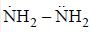having lower activation energy so they act as more nucleophile.

*Answer can only contain numeric values
QUESTION: 19

x = number of (+M) group attached with phenyl ring, so the value of x is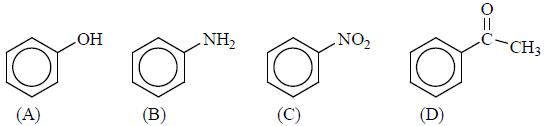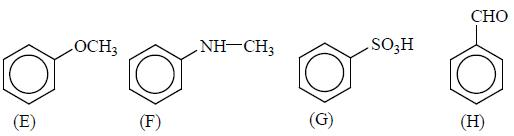Solution:

Lone pair- containing atom with conjugation show (+M) effect.
A, B, E, F ( + M ) effect
C, D, G, H ( - M ) effect

*Answer can only contain numeric values
QUESTION: 20

Number of non-aromatic compound given below.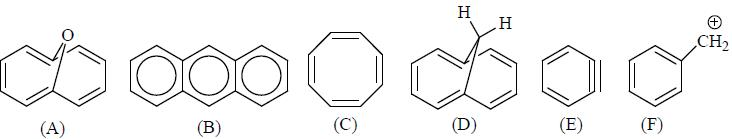Solution:

A → Aromatic
B → Aromatic
C → Non-aromatic
D → Aromatic
E → Aromatic
D → Aromatic

Track your progress, build streaks, highlight & save important lessons and more!

### Similar Content### Related tests